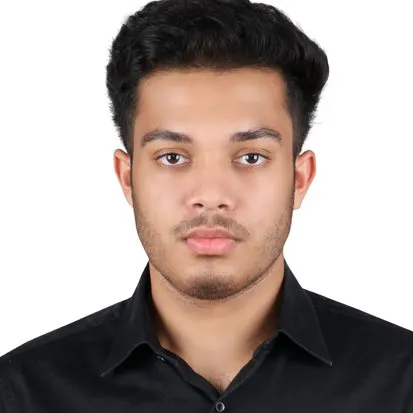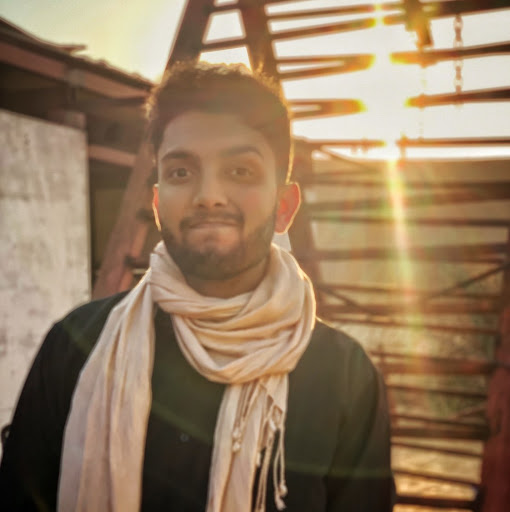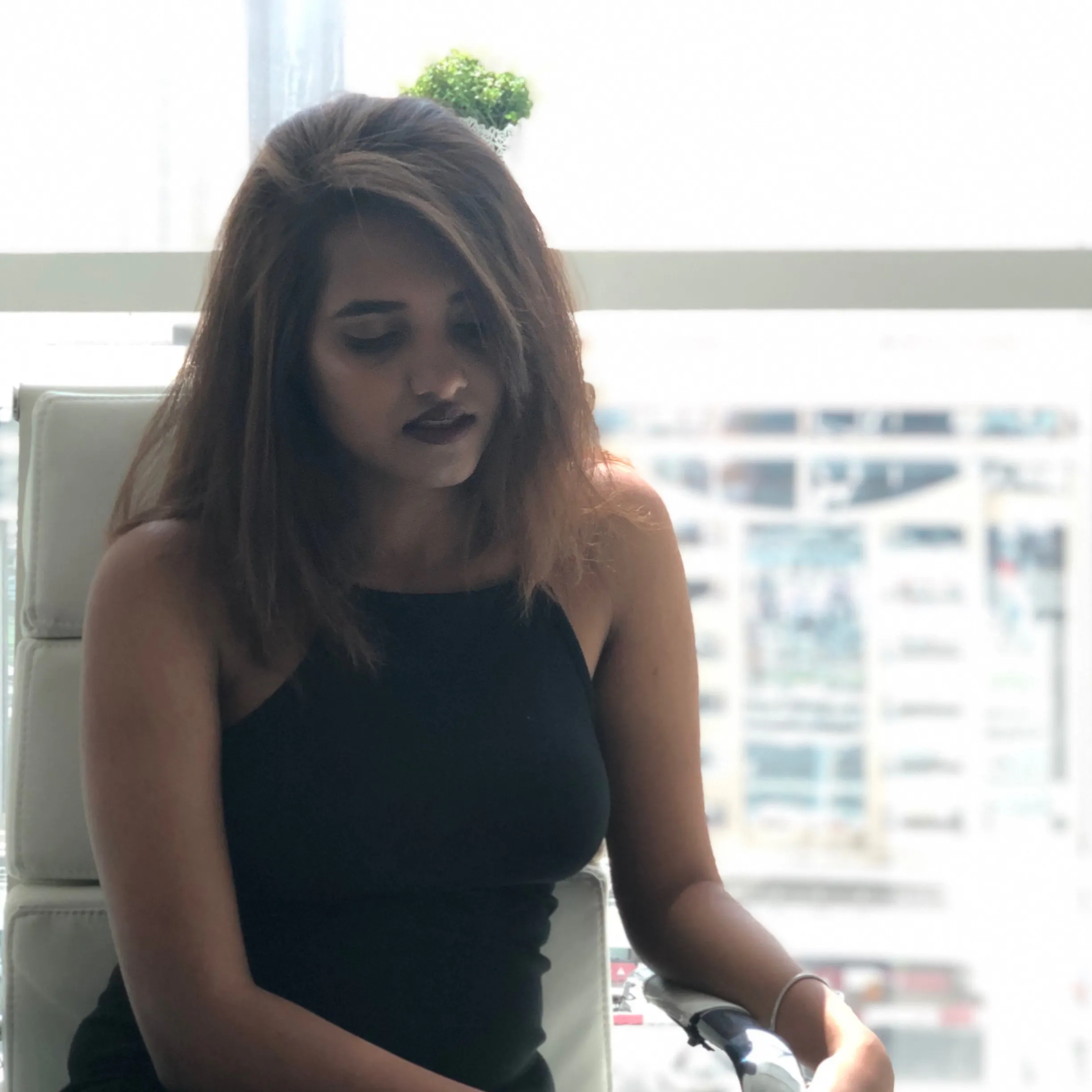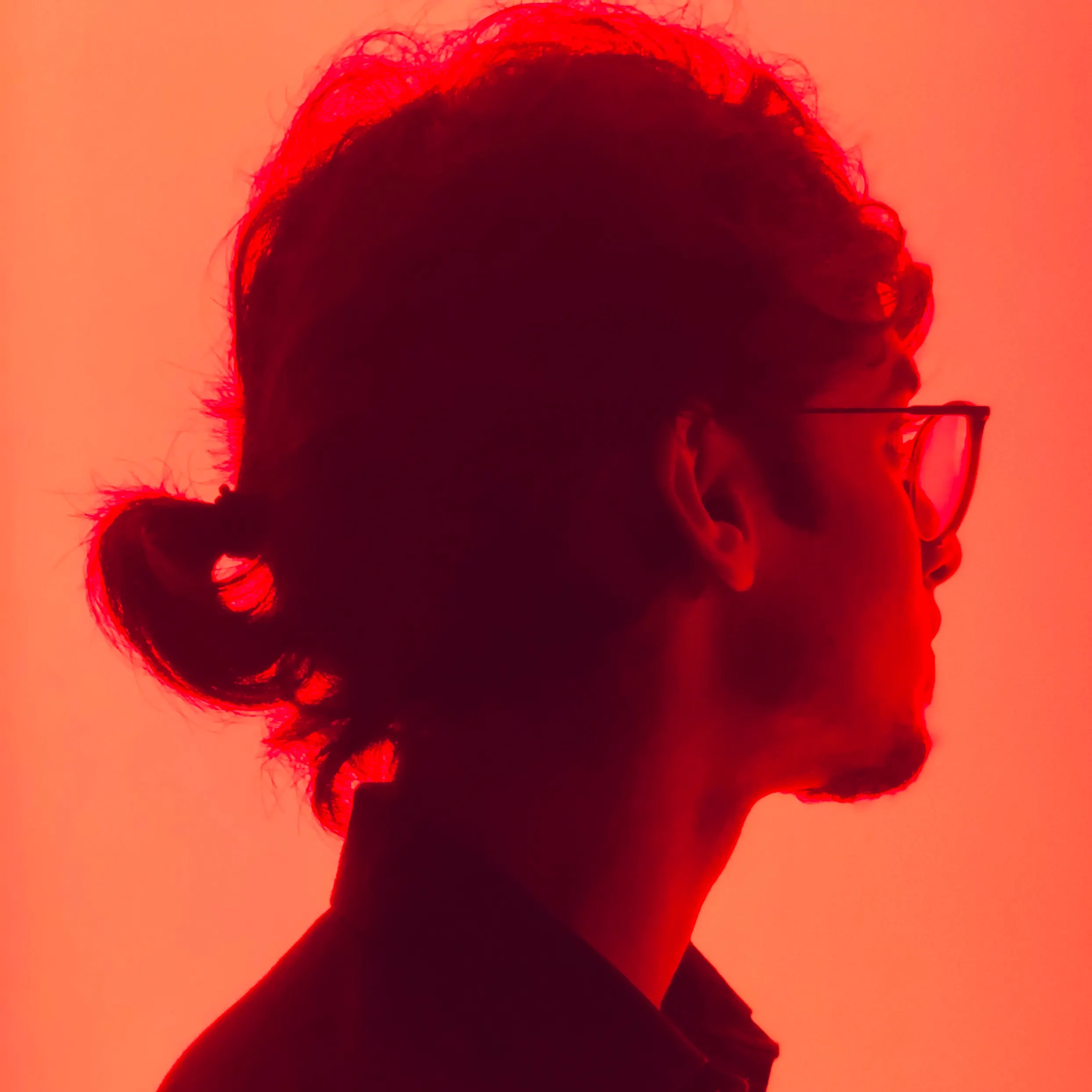# Fluoresce Robotics - Autonomous UVC Disinfection Robot

The Fluoresce Robot is an autonomous disinfection robot which uses UVC radiation to provide a germ-free environment.

## Things used in this project

### Hardware components

 NVIDIA Jetson AGX Xavier Developer Kit Main processing unit of the Robot. ROS is running on this device and assists with all the decision making for the movement and control of the robot.
×1
 ZED Stereo Camera Front-facing Stereo Vision Camera for Object and Person Detection, as well as Navigation using SLAM
×1
 Slamtec RPLIDAR A1 360 degree LIDAR for SLAM, integration with ROS
×1
 Philips TUV 180W XPT SE UNP/20 \$235/pc: UVC Light, 180W, Dimmable, Single Ended, Universal Operating Position
×4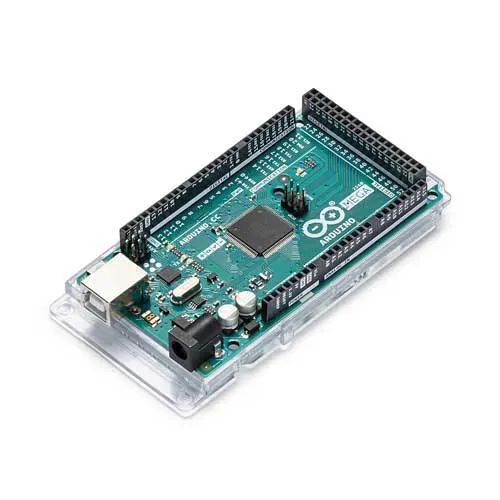Arduino Mega 2560 Control of UV Tube servos. wheels and other various actuators.
×1
 Cleaning indicator LED strip (1.178m ) Safety lights placed on the bottom of the robot which indicates if the robot is safe to operate.
×1
 Cleaning indicator LED strip (0.549m) Safety lights placed on the top of the robot which indicates if the robot is safe to operate.
×1
 Intel wifi module
×1

### Software apps and online servicesROS Robot Operating System Main software used for control and manipulation of the robot.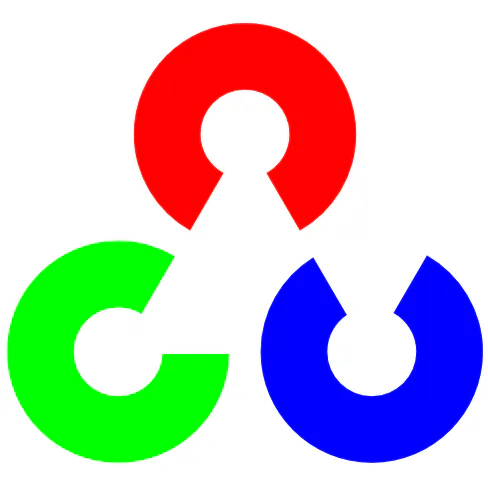OpenCV Software used to perform active Computer Vision tasks like detecting presence of Humans/Animals as well as detection of other high risk surfaces.Arduino IDE Application used to code to control various components like servos and wheels of the Robot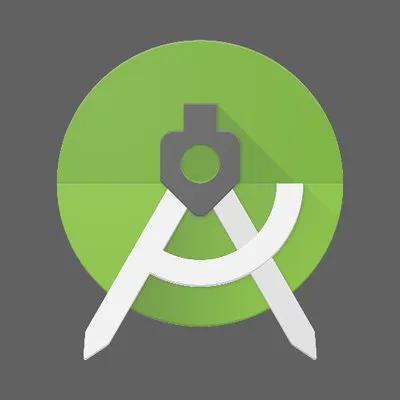Android Studio
 Blender Application used to design all the animations of Robot features and movements.
 Adobe XD Application used for designing a prospective User Interface for Robot control and monitoring.
 Shapr3D Application used for designing the Robot 3D CAD Models.
 Procreate® Application used for creating the Cover Image and other illustrations and diagrams.

### Hand tools and fabrication machines3D Printer (generic)Soldering iron (generic)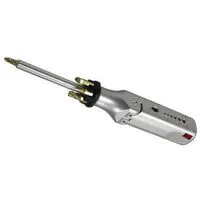Multitool, Screwdriver
 Hexagonal screws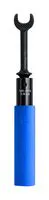Torque Wrench, 7/16 " Drive

## Custom parts and enclosures

### Exploded View of the Robot

It depicts all the hardware components that make up the robot.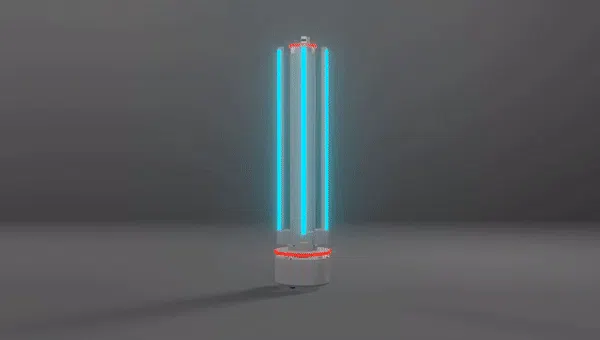### Tube Mechanism - Exploded View

This is the mechanism used to allow the tube to be rotated from 0-90degree with the help of a servo and a few gears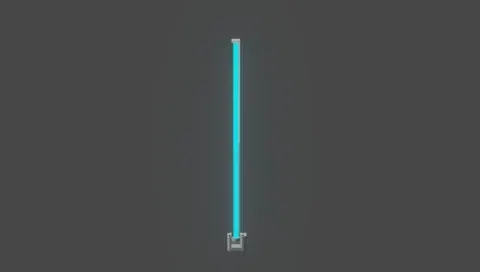## Schematics

### Power supply system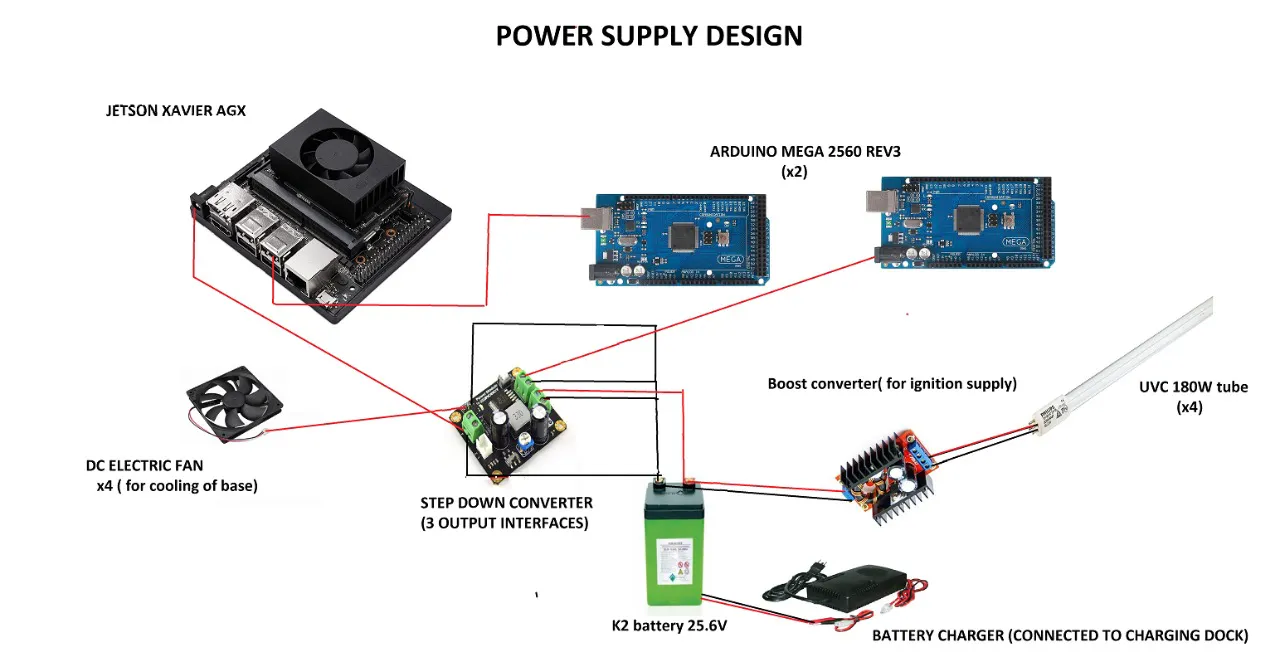## Code

### object_detection_ros.py

Python
It is a subscriber program which gets a camera image from the publisher and converts it into an OpenCV compatible format so as to look for different objects.
```#Subscriber program to detect an object

#Import the required packages
import rospy
import cv2
import numpy as np
import imutils
import matplotlib.pyplot as plt
import cvlib as cv
from std_msgs.msg import String
from sensor_msgs.msg import Image
from cv_bridge import CvBridge, CvBridgeError
from cvlib.object_detection import draw_bbox
import sys

bridge = CvBridge()

def image_callback(ros_image):
print ('Got an image')
global bridge

#convert ros_image into an opencv-compatible image
try:
cv_image = bridge.imgmsg_to_cv2(ros_image, "bgr8")
except CvBridgeError as e:
print(e)

#Detecting an object
bbox, label, conf = cv.detect_common_objects(cv_image)
output_image = draw_bbox(cv_image, bbox, label, conf)
cv2.imshow('output_image', cv_image)

#Stopping Program if living being detected
if 'person' in label or 'potted plant' in label or 'pet' in label:
break

def main(args):
rospy.init_node('image_converter', anonymous=True)
#image_topic="/usb_cam/image_raw"
image_sub = rospy.Subscriber("/usb_cam/image_raw",Image, image_callback)
try:
rospy.spin()
except KeyboardInterrupt:
print("Shutting down")
cv2.destroyAllWindows()

if __name__ == '__main__':
main(sys.argv)

```

### people_detect_ros.py

Python
Program to ensure the room is empty before the sanitization process begins.
```#Subscriber program to detect a person

#Import the required packages
import rospy
import numpy as np
import imutils
import cv2
from std_msgs.msg import String
from sensor_msgs.msg import Image
from cv_bridge import CvBridge, CvBridgeError
import sys

bridge = CvBridge()

# initialize the HOG descriptor/person detector
hog = cv2.HOGDescriptor()
hog.setSVMDetector(cv2.HOGDescriptor_getDefaultPeopleDetector())

def image_callback(ros_image):
print ('Got an image')
global bridge

#convert ros_image into an opencv-compatible image
try:
cv_image = bridge.imgmsg_to_cv2(ros_image, "bgr8")
except CvBridgeError as e:
print(e)

#detecting people in the image
(rects, weights) = hog.detectMultiScale(cv_image, winStride=(4, 4),padding=(4,4), scale=1.01)

#draw the bounding boxes
for (x, y, w, h) in rects:
cv2.rectangle(image, (x, y), (x + w, y + h), (0, 0, 255), 2)

cv2.imshow('Image', cv_image)

if (any(weights)):
print ("PERSON DETECTED")
break

def main(args):
rospy.init_node('image_converter', anonymous=True)
#image_topic="/usb_cam/image_raw"
image_sub = rospy.Subscriber("/usb_cam/image_raw",Image, image_callback)
try:
rospy.spin()
except KeyboardInterrupt:
print("Shutting down")
cv2.destroyAllWindows()

if __name__ == '__main__':
main(sys.argv)
```

### activity_main.xml

XML
Code for Android Studio for one of the screens of the application.
```<?xml version="1.0" encoding="utf-8"?>
<androidx.constraintlayout.widget.ConstraintLayout xmlns:android="http://schemas.android.com/apk/res/android"
xmlns:app="http://schemas.android.com/apk/res-auto"
xmlns:tools="http://schemas.android.com/tools"
android:layout_width="match_parent"
android:layout_height="match_parent"
tools:context=".MainActivity"
>

<ImageView
android:layout_width="581dp"
android:layout_height="915dp"
android:scaleType="centerCrop"
android:src="@drawable/design"
app:layout_constraintBottom_toBottomOf="parent"
app:layout_constraintEnd_toEndOf="parent"
app:layout_constraintStart_toStartOf="parent"
app:layout_constraintTop_toTopOf="parent"
app:layout_constraintVertical_bias="0.069" ></ImageView>

<EditText
android:id="@+id/editTextTextPersonName"
android:layout_width="97dp"
android:layout_height="23dp"
android:ems="20"
android:inputType="textPersonName"
android:text="Robot Status"
app:layout_constraintBottom_toBottomOf="parent"
app:layout_constraintEnd_toEndOf="parent"
app:layout_constraintHorizontal_bias="0.226"
app:layout_constraintStart_toStartOf="parent"
app:layout_constraintTop_toTopOf="parent"
app:layout_constraintVertical_bias="0.216"
android:textSize= "15sp" ></EditText>

<EditText
android:id="@+id/editTextTextPersonName2"
android:layout_width="122dp"
android:layout_height="33dp"
android:ems="10"
android:inputType="textPersonName"
android:text="Room Clean"
android:textSize="20sp"
app:layout_constraintBottom_toBottomOf="parent"
app:layout_constraintEnd_toEndOf="parent"
app:layout_constraintHorizontal_bias="0.808"
app:layout_constraintStart_toStartOf="parent"
app:layout_constraintTop_toTopOf="parent"
app:layout_constraintVertical_bias="0.219" ></EditText>

<EditText
android:id="@+id/editTextTextPersonName3"
android:layout_width="wrap_content"
android:layout_height="wrap_content"
android:ems="10"
android:inputType="textPersonName"
android:text="Select Room"
android:textSize="20sp"
app:layout_constraintBottom_toBottomOf="parent"
app:layout_constraintEnd_toEndOf="parent"
app:layout_constraintHorizontal_bias="0.437"
app:layout_constraintStart_toStartOf="parent"
app:layout_constraintTop_toTopOf="parent"
app:layout_constraintVertical_bias="0.358" ></EditText>

<EditText
android:id="@+id/editTextTextPersonName4"
android:layout_width="200dp"
android:layout_height="26dp"
android:ems="10"
android:inputType="textPersonName"
android:text="Robot temperature"
android:textSize="20sp"
app:layout_constraintBottom_toBottomOf="parent"
app:layout_constraintEnd_toEndOf="parent"
app:layout_constraintHorizontal_bias="0.362"
app:layout_constraintStart_toStartOf="parent"
app:layout_constraintTop_toTopOf="parent"
app:layout_constraintVertical_bias="0.476" ></EditText>

<EditText
android:id="@+id/editTextTextPersonName5"
android:layout_width="wrap_content"
android:layout_height="wrap_content"
android:ems="10"
android:inputType="textPersonName"
android:text="Battery Level"
android:textSize="20sp"
app:layout_constraintBottom_toBottomOf="parent"
app:layout_constraintEnd_toEndOf="parent"
app:layout_constraintHorizontal_bias="0.437"
app:layout_constraintStart_toStartOf="parent"
app:layout_constraintTop_toTopOf="parent"
app:layout_constraintVertical_bias="0.62" ></EditText>

<EditText
android:id="@+id/editTextTextPersonName6"
android:layout_width="wrap_content"
android:layout_height="wrap_content"
android:ems="10"
android:inputType="textPersonName"
android:text="Cleaning Progress"
android:textSize="20sp"
app:layout_constraintBottom_toBottomOf="parent"
app:layout_constraintEnd_toEndOf="parent"
app:layout_constraintHorizontal_bias="0.437"
app:layout_constraintStart_toStartOf="parent"
app:layout_constraintTop_toTopOf="parent"
app:layout_constraintVertical_bias="0.819" ></EditText>

<EditText
android:id="@+id/editTextNumber2"
android:layout_width="wrap_content"
android:layout_height="wrap_content"
android:ems="10"
android:inputType="number"
app:layout_constraintEnd_toEndOf="parent"
app:layout_constraintHorizontal_bias="0.786"
app:layout_constraintStart_toStartOf="parent"
app:layout_constraintTop_toTopOf="parent"
android:textSize= "20sp">

<EditText
android:id="@+id/editTextTextPersonName7"
android:layout_width="60dp"
android:layout_height="22dp"
android:layout_marginBottom="368dp"
android:ems="10"
android:inputType="textPersonName"
android:text="50C"
android:textSize="20sp"
app:layout_constraintBottom_toBottomOf="parent"
app:layout_constraintEnd_toEndOf="parent"
app:layout_constraintHorizontal_bias="0.21"
app:layout_constraintStart_toStartOf="parent" ></EditText>

<EditText
android:id="@+id/editTextTextPersonName8"
android:layout_width="53dp"
android:layout_height="19dp"
android:ems="10"
android:inputType="textPersonName"
android:text="80"
android:textSize="20sp"
app:layout_constraintBottom_toBottomOf="parent"
app:layout_constraintEnd_toEndOf="parent"
app:layout_constraintHorizontal_bias="0.826"
app:layout_constraintStart_toStartOf="parent"
app:layout_constraintTop_toTopOf="parent"
app:layout_constraintVertical_bias="0.624" ></EditText>

<EditText
android:id="@+id/editTextTextPersonName9"
android:layout_width="48dp"
android:layout_height="35dp"
android:ems="10"
android:inputType="textPersonName"
android:text="30"
android:textSize="20sp"
app:layout_constraintBottom_toBottomOf="parent"
app:layout_constraintEnd_toEndOf="parent"
app:layout_constraintHorizontal_bias="0.788"
app:layout_constraintStart_toStartOf="parent"
app:layout_constraintTop_toTopOf="parent"
app:layout_constraintVertical_bias="0.836" ></EditText>

android:layout_width="13dp"
android:layout_height="17dp"
app:layout_constraintBottom_toBottomOf="parent"
app:layout_constraintEnd_toEndOf="parent"
app:layout_constraintHorizontal_bias="0.702"
app:layout_constraintStart_toStartOf="parent"
app:layout_constraintTop_toTopOf="parent"

android:layout_width="wrap_content"
android:layout_height="wrap_content"
app:layout_constraintBottom_toBottomOf="parent"
app:layout_constraintEnd_toEndOf="parent"
app:layout_constraintHorizontal_bias="0.302"
app:layout_constraintStart_toStartOf="parent"

</androidx.constraintlayout.widget.ConstraintLayout>
```

### Mapping_the_room.py

Python
Program to map the room on a graph based on inputs from the robot..
```from matplotlib import pyplot as plt

def getPoint(c):
global x, y, flag
if (c=='R' or c=='r'):
flag+=1
elif (c=='L' or c=='l') :
flag-=1
flag = flag % 4

dist = int(input ("Distance : "))
if flag == 0:
y+=dist
elif flag == 1:
x+=dist
elif flag == 2:
y-=dist
elif flag == 3:
x-=dist
points.append ((x,y))
X.append (x)
Y.append (y)

x=y=0
X=[]
Y=[]
flag = 0
#0:forward; 1:right; 2:backwards; 3:left
points = []
points.append((x,y))
X.append (x)
Y.append (y)

c = input("Left/Right/Foward? ")
getPoint(c)

while (x!=0 or y!=0):
c = input("Left/Right? ")
getPoint(c)

print (X,Y)
plt.plot(X,Y, scalex=True, scaley=True)
plt.show()

```

### Obstacle_Detection

Python
Program used to steer the robot away from obstacles.
```#!/usr/bin/env python

from __future__ import print_function

import rospy

from std_msgs.msg import Float64
from sensor_msgs.msg import LaserScan

import sys, select, termios, tty

msg = """
---------------------------
Moving around:
u    i    o
j    k    l
m    ,    .

For Holonomic mode (strafing), hold down the shift key:
---------------------------
U    I    O
J    K    L
M    <    >

anything else : stop

q/z : increase/decrease max speeds by 10%

CTRL-C to quit
"""

moveBindings = {
'i':(-1,0,1),
'o':(-1,1,0),
'j':(1,1,1),
'l':(-1,-1,-1),
'u':(-1,0,1),
',':(1,0,-1),
'.':(0,1,-1),
'm':(1,-1,0),
'O':(-1,1,0),
'I':(-1,0,1),
'J':(1,-2,1),
'L':(-1,2,-1),
'U':(0,-1,1),
'<':(1,0,-1),
'>':(0,1,-1),
'M':(1,-1,0),
}

speedBindings={
'q':(1.1,1.1),
'z':(.9,.9),
}

def vels(speed):
return "currently:\tspeed %s " % (speed)

pub = None

def clbk_laser(msg):
regions = {
'r' : min(min(msg.ranges[0:78]),20),
'fr' : min(min(msg.ranges[102:180]),20),
'fl' : min(min(msg.ranges[182:258]),20),
'l' : min(min(msg.ranges[282:360]),20),
'bl' : min(min(msg.ranges[362:438]),20),
'br' : min(min(msg.ranges[642:718]),20)
}

take_action(regions)

def take_action(regions):
msg = Twist()

state_description = ''

if regions['l']>1 and regions['fl']>1 and regions['fr']>1 and regions['r']>1:
state_description = 'case 1 - nothing'
key='i'
elif regions['l']>1 and regions['fl']>1 and regions['fr']>1 and regions['r']<1:
state_description = 'case 2 - right'
key='j'

elif regions['l']>1 and regions['fl']>1 and regions['fr']<1 and regions['r']>1:
state_description = 'case 3 - front right'
key='j'

elif regions['l']>1 and regions['fl']>1 and regions['fr']<1 and regions['r']<1:
state_description = 'case 4 - front right and right'
key='j'

elif regions['l']>1 and regions['fl']<1 and regions['fr']>1 and regions['r']>1:
state_description = 'case 5 - front left'
key='l'

elif regions['l']>1 and regions['fl']<1 and regions['fr']>1 and regions['r']<1:
state_description = 'case 6 - front left and right'
key='o'

elif regions['l']>1 and regions['fl']<1 and regions['fr']<1 and regions['r']>1:
state_description = 'case 7 - front right and front left'
key='l'

elif regions['l']>1 and regions['fl']<1 and regions['fr']<1 and regions['r']<1:
state_description = 'case 8 - front left, front right and right'
key='j'

elif regions['l']<1 and regions['fl']>1 and regions['fr']>1 and regions['r']>1:
state_description = 'case 9 - left'
key='l'

elif regions['l']<1 and regions['fl']>1 and regions['fr']>1 and regions['r']<1:
state_description = 'case 10 - left and right'
key='o'

elif regions['l']<1 and regions['fl']>1 and regions['fr']<1 and regions['r']>1:
state_description = 'case 11 - left and front right'
key='l'

elif regions['l']<1 and regions['fl']>1 and regions['fr']<1 and regions['r']<1:
state_description = 'case 12 - left, front right and right'
key='u'

elif regions['l']<1 and regions['fl']<1 and regions['fr']>1 and regions['r']>1:
state_description = 'case 13 - left and front left'
key='l'

elif regions['l']<1 and regions['fl']<1 and regions['fr']>1 and regions['r']<1:
state_description = 'case 14 - left, front left and right'
key='o'
elif regions['l']<1 and regions['fl']<1 and regions['fr']<1 and regions['r']>1:
state_description = 'case 15-left, front left and front right'
key='l'
else:
state_description = 'case 16 - All directions'
key='m'

return key
pub.publish(msg)

def main():
global pub

sub = rospy.Subscriber('/uvrobot/laser/scan', LaserScan, clbk_laser)

rospy.spin()

if __name__=="__main__":
settings = termios.tcgetattr(sys.stdin)

rospy.init_node('vel_Publisher')
publ = rospy.Publisher('/open_base/left_joint_velocity_controller/command', Float64, queue_size=1)
pubb = rospy.Publisher('/open_base/back_joint_velocity_controller/command', Float64, queue_size=1)
pubr = rospy.Publisher('/open_base/right_joint_velocity_controller/command', Float64, queue_size=1)

speed = 1.0
x = 0
y = 0
z = 0
status = 0

try:
print(msg)
print(vels(speed))
while(1):
key = take_action(regions)
if key in moveBindings.keys():
x = moveBindings[key]
y = moveBindings[key]
z = moveBindings[key]
elif key in speedBindings.keys():
speed = speed * speedBindings[key]

print(vels(speed))
if (status == 14):
print(msg)
status = (status + 1) % 15
else:
x = 0
y = 0
z = 0
th = 0
if (key == '\x03'):
break

vell = Float64()
velb = Float64()
velr = Float64()

vell = x*speed
velb = y*speed
velr = z*speed

publ.publish(vell)
pubb.publish(velb)
pubr.publish(velr)

except Exception as e:
print(e)

finally:
vell = Float64()
velb = Float64()
velr = Float64()

vell = 0.0
velb = 0.0
velr = 0.0

pubb.publish(vell)
publ.publish(velb)
pubr.publish(velr)
```

## Credits

### Kevin R

3 projects • 0 followers
Aerospace Enthusiast | Senior Year of Electrical and Electronic Engineering at BITS Pilani, Dubai

### Nikhil Binu Thomas

1 project • 0 followers

### Clayton Pereira

1 project • 0 followers
Senior Year Electronics and Communication Student at BITS Pilani, Dubai.

### Tanya Mathur

1 project • 0 followers

### Nikita Kulkarni

1 project • 0 followers
Final Year Biotechnology Student

### Suhail Nazeer

1 project • 0 followers
Final Year Electrical & Electronics Engineering
Thanks to Yug Ajmera.